# Electric Field Enhancement Equations

2 Parallel Plates
Sphere/ Plate
2 Spheres
Concentric Spheres
Cylinder / Plate
Parallel Cylinders
Perpendicular Cylinders
Coaxial Cylinders
Hemisphere/ Plate
Semicylinder / Plate
2 Dielectrics

The formulas on this page allow one to calculate the maximum electric fields (or electric field enhancement factors) for certain given typical geometries.  More complex problems normally require one to model the geometry and solve using Finite Element Analysis. The average electric field in a gap between electrodes is the potential difference divided by the minimum electrode separation. The field enhancement factor is then defined as the maximum electric field divided by the average electric field. The field enhancement factor of 1.0 therefore represents no enhancement over the average field.

These formulas were originally published in the following document: “The Maximum Electric Field Strength for Several Simple Electrode Configurations”, A.B. Bowers and P.G. Cath, Phillips Tech. Rev. 6, #270 (1941).

### 2 Parallel Plane PlatesParallel Plate Geometry for E-Field Calculation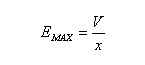Equation for Parallel Plate Electric Fields V is the Voltage applied x is the distance between the plates

The graph below shows an electric field plot between a pair of parallel plates where one plate has a voltage of 1000 V and the other plate is held at ground potential. The plates are spaced 1 cm apart and the model is plane/parallel where the features extend into the model plane (the z direction) with the same x and y dimensions. The calculated maximum field is 100 kV/m according to the equation above while the results from the Efield plotting software is shown as 100 kV/m below. The color map shows electrical field strength while the black lines show equipotential lines at 50 V increments. As one can see, the electric field strength is uniform in the gap between the two plates and the field enhancement factor is equal to 1.0.

### Sphere and Plane Plate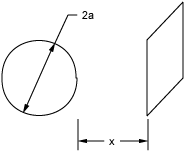Diagram For Field Enhancement or Maximum Electric Field Between Sphere and Plate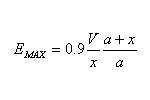Equation For Field Enhancement or Maximum Electric Field Between Sphere and Plate V is the Voltage applied x is the distance between the sphere and the plane plate a is the radius of the sphere

The graph below shows an electric field plot between a sphere and a plane where the sphere has a voltage of 1000 V and the plane is held at ground potential. The sphere is spaced 6 mm apart from the plane and has a 3 mm radius. In this case, the model is axi-symmetric where the horizontal axis is the z direction and the vertical axis is the radial direction. All features are then rotated around the horizontal axis. The calculated maximum field is 450 kV/m according to the equation above while the results from the Efield plotting software is shown as 426 kV/m below (a difference of less than 5%). The color map shows electrical field strength while the black lines show equipotential lines at 50 V increments. In this case, the field enhancement factor is equal to 2.7.

### 2 SpheresDiagram For Field Enhancement or Maximum Electric Field Between Two SpheresEquation For Field Enhancement or Maximum Electric Field Between Two Spheres V is the Voltage applied x is the distance between the two spheres a is the radius of each sphere

The graph below shows an electric field plot between a pair of two spheres where one sphere has a voltage of 1000 V and the other is held at ground potential. The spheres are spaced 6 mm apart and each has a 3 mm radius. As with the previous sphere problem, this model is axi-symmetric and the model features rotate around the horizontal axis. The calculated maximum field is 300 kV/m according to the equation above while the results from the Efield plotting software is shown as 291 kV/m below (a difference of less than 3%). The color map shows electrical field strength while the black lines show equipotential lines at 50 V increments. The field enhancement factor for this geometry is equal to 1.8.

### 2 Concentric Spheres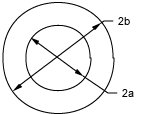Diagram For Field Enhancement or Maximum Electric Field Between Two Concentric Spheres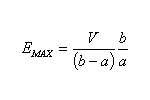Equation For Field Enhancement or Maximum Electric Field Between Two Concentric Spheres V is the Voltage applied a is the radius of the inner sphere b is the radius of the outer sphere

The graph below shows an electric field plot of a pair of concentric spheres where the inner sphere has a voltage of 1000 V and the outer sphere is held at ground potential. The inner sphere has a radius of 3 mm (0.003 m) and the radius of the outer sphere is 9 mm (0.009 m). Once again, the model is axi-symmetric. The calculated maximum field is 500 kV/m according to the equation above while the results from the Efield plotting software is shown as 496.1 kV/m below (a difference of less than 1%). The color map shows electrical field strength while the black lines show equipotential lines at 50 V increments. This specific example defines a field enhancement factor of 3.0.

### Cylinder and PlateDiagram For Field Enhancement or Maximum Electric Field Between a Cylinder and a Plate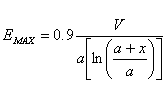Equation For Field Enhancement or Maximum Electric Field Between a Cylinder and a Plate V is the Voltage applied x is the distance between the cylinder and the plane plate a is the radius of the cylinder

The graph below shows an electric field plot of a cylinder and a plate where the cylinder has a voltage of 1000 V and the plate is held at ground potential. The cylinder in this plane/parallel model has a radius of 3 mm (0.003 m) and the distance between the cylinder and plate is 6 mm (0.006 m). The calculated maximum field is 273.1 kV/m according to the equation above while the results from the Efield plotting software is shown as 266 kV/m below (a difference of ~2.5%). The color map shows electrical field strength while the black lines show equipotential lines at 50 V increments. The field enhancement factor for this example is approximately 1.64.

### 2 Parallel Cylinders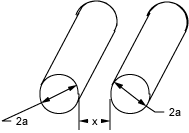Diagram For Field Enhancement or Maximum Electric Field Between Two Parallel CylindersEquation For Field Enhancement or Maximum Electric Field Between Two Parallel Cylinders V is the Voltage applied x is the distance between the two cylinders a is the radius of each cylinder

The graph below shows an electric field plot of two parallel cylinders where the left one has a voltage of 1000 V and the right one is held at ground potential. Each cylinder in the plane/parallel model has a radius of 3 mm (0.003 m) and the distance between the two cylinders is 6 mm (0.006 m). The calculated maximum field is 216.4 kV/m according to the equation above while the results from the Efield plotting software is shown as 215 kV/m below (a difference of ~1%). The color map shows electrical field strength while the black lines show equipotential lines at 50 V increments. In this case, the field enhancement factor is approximately 1.30.

### 2 Perpendicular CylindersDiagram For Field Enhancement or Maximum Electric Field Between Two Perpendicular Cylinders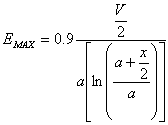Equation For Field Enhancement or Maximum Electric Field Between Two Perpendicular Cylinders V is the Voltage applied x is the distance between the two cylinders a is the radius of each cylinder

### 2 Coaxial Cylinders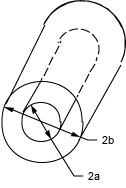Diagram For Field Enhancement or Maximum Electric Field Between Two Concentric CylindersEquation For Field Enhancement or Maximum Electric Field Between Two Concentric Cylinders V is the Voltage applied a is the radius of the inner cylinder b is the radius of the outer cylinder

The graph below shows an electric field plot of two concentric (coaxial) cylinders where the inner cylinder has a voltage of 1000 V and the outer cylinder is held at ground potential. The inner cylinder has a radius of 3 mm and the outer cylinder has a radius of 9 mm. In this case, only a quarter section has been modeled to reduce the computational time since the plane/parallel model is symmetric. The calculated maximum field is 303.4 kV/m according to the equation above while the results from the Efield plotting software is shown as 303 kV/m below (a difference of less than 1%). The color map shows electrical field strength while the black lines show equipotential lines at 50 V increments. This geometry has a field enhancement factor of 1.82.

The graph below shows an electric field plot of RG-220 coaxial cable where the center conductor is at a 35 kV potential and the outer braid is at ground. The calculated maximum field is 8.461 MV/m according to the equation above while the results from the Efield plotting software is shown as 8.490 MV/m below (a difference of less than 1%). The color map shows electrical field strength while the black lines show equipotential lines at 2500 V increments. This field level equates to ~215 V/mil compared to the breakdown strength of polyethylene of ~450-550 V/mil. One can also see from the plot that the fields at the outside diameter of the polyethylene are almost 3 MV/m which is approximately the breakdown strength of air at sea level. If any air gaps exist between the insulation and the braid (or if the braid has any broken strands that might provide a field enhancement), the fields may be strong enough to create a corona discharge in that region.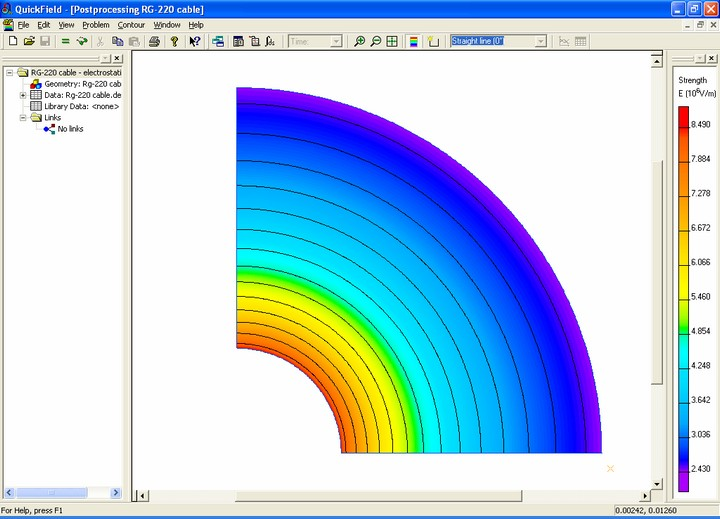Graph or Plot of Electric Fields in RG-220 Coaxial Cable at 35 kV

### Hemisphere on 1 of 2 Parallel Planes (x >> a)Diagram For Field Enhancement or Maximum Electric Field Between a Hemisphere and a Plate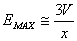Equation For Field Enhancement or Maximum Electric Field Between a Hemisphere and a Plate V is the Voltage applied x is the distance between the two plane plates a is the radius of the hemisphere

The graph below shows an electric field plot of two parallel plates where the left one has a voltage of 1000 V and the right one is held at ground potential. The left plate has a hemisphere on it which has a radius of 3 mm (0.003 m) and the distance between the two plates is 33 mm (0.033 m). The calculated maximum field is 90.9 kV/m according to the equation above while the results from the Efield plotting software is shown as 89.8 kV/m below (a difference of ~1%). The color map shows electrical field strength. In this case, the field enhancement factor is approximately 3.

### Semi-cylinder on 1 of 2 Parallel Plane Plates (x >> r)Diagram For Field Enhancement or Maximum Electric Field Between a Semi-Cylinder and a Plate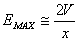Equation For Field Enhancement or Maximum Electric Field Between a Semi-Cylinder and a Plate V is the Voltage applied x is the distance between the two plane plates r is the radius of the cylinder

The graph below shows an electric field plot of two parallel plates where the left one has a voltage of 1000 V and the right one is held at ground potential. The left plate has a semi-cylinder on it which has a radius of 3 mm (0.003 m) and the distance between the two plates is 36 mm (0.033 m). The calculated maximum field is 55.6 kV/m according to the equation above while the results from the Efield plotting software is shown as 55.2 kV/m below (a difference of less than 1%). The color map shows electrical field strength. In this case, the field enhancement factor is approximately 2.

### Two Dielectrics Between Plane Plates (x1 > x2)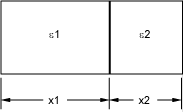Diagram For Field Enhancement or Maximum Electric Field Between Two Different Dielectric Materials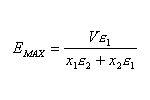Equation For Field Enhancement or Maximum Electric Field Between Two Different Dielectric Materials V is the Voltage applied x1 is the thickness of the 1st dielectric x2 is the thickness of the 2nd dielectric e1 is the dielectric constant of the 1st dielectric e2 is the dielectric constant of the 2nd dielectric

The graph below shows an electric field plot of two different dielectric materials in series between a pair of parallel plates where one plate has a voltage of 1000 V and the other plate is held at ground potential. The thicker slab of dielectric in this plane/parallel model is 0.8 cm thick and has a (relative) dielectric constant of 2. The thinner slab of dielectric is 0.2 cm thick and has a dielectric constant of 1. The calculated maximum field is 166.6 kV/m according to the equation above while the results from the Efield plotting software is shown as 167 kV/m below (a difference of less than 1%). The color map shows electrical field strength while the black lines show equipotential lines at 50 V increments. One can see that the fields are concentrated in the material with the lower dielectric constant. The fields in the higher dielectric slab are only 83.3 kV/m. Compare these results to the first example at the top of this page where the electric field throughout the area between the two electrodes is 100 kV/m. The lower dielectric material has a field enhancement factor of 1.67.Graph or Plot of Electric Fields Between Two Layers of Different Dielectric Materials